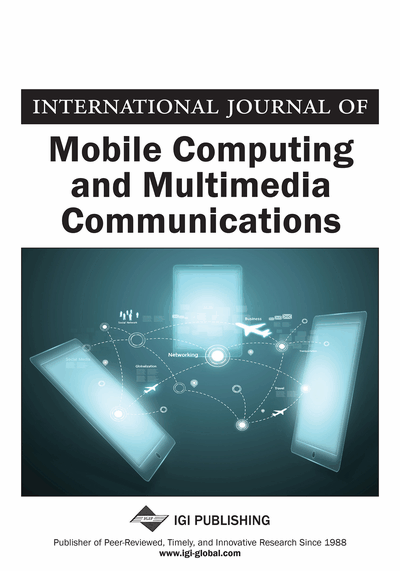# Multi-Agent Actor Critic for Channel Allocation in Heterogeneous Networks

Nan Zhao (Hubei University of Technology, China), Zehua Liu (Hubei University of Technology, China), Yiqiang Cheng (Hubei University of Technology, China) and Chao Tian (Hubei University of Technology, China)
DOI: 10.4018/IJMCMC.2020010102

## Abstract

Heterogeneous networks (HetNets) can equalize traffic loads and cut down the cost of deploying cells. Thus, it is regarded to be the significant technique of the next-generation communication networks. Due to the non-convexity nature of the channel allocation problem in HetNets, it is difficult to design an optimal approach for allocating channels. To ensure the user quality of service as well as the long-term total network utility, this article proposes a new method through utilizing multi-agent reinforcement learning. Moreover, for the purpose of solving computational complexity problem caused by the large action space, deep reinforcement learning is put forward to learn optimal policy. A nearly-optimal solution with high efficiency and rapid convergence speed could be obtained by this learning method. Simulation results reveal that this new method has the best performance than other methods.
Article Preview
Top

## Introduction

With the number of wireless devices increasing fast, mobile communication networks (Kaushik et al., 2019; Yue et al., 2019) are confronting the enormous challenge of increasing network capacity (Huang et al., 2017; Zhao et al., 2017; Zhao et al., 2019a). Densifying existing cells using the pico base station (PBS) with different transmit power and coverage is an effective solution. Heterogeneous networks (HetNets) (Xia et al., 2018; Helmy et al., 2018; Alhabo et al., 2019) make the service provider possible to unload the user equipment (UE) from the macro base station (MBS) to the PBS, which can not only balance traffic load but also cut down the cost of deploying cells (Wu et al., 2018; Papazafeiropoulos et al., 2018). Furthermore, since the same channel can be shared by PBSs, the overall spectrum efficiency of cellular networks can be improved accordingly (Zhang et al., 2018; Panahi et al., 2018;). Therefore, HetNets have been considered as an effective approach to increase the network capacity and energy efficiency of cellular networks.

Many performance optimization issues can be seen in HetNets (Zhao et al., 2019b), in which channel allocation is a common problem (Dao et al., 2018; Xu et al., 2018). Channel allocation was considered as an important measure to solve the issue of load balancing in HetNets. In (Zhao et al., 2018a; Wang et al., 2018; Wang et al., 2018), authors investigated the problem of channel allocation. However, the optimization problem has the non-convex features, obtaining a globally optimal strategy is very difficult. Many new methods have been developed to solve these problems, such as Markov approximation (Chen et al., 2013), game-theoretic approach (Zhang et al., 2018) and linear programming method (Elsherif et al., 2015). Almost accurate and complete network information are required to effectively obtain the optimal strategies for these methods. However, it is hard to achieve complete information, which makes the calculation of the optimal maneuver intractable to handle. In this paper, reinforcement learning (RL) is deployed in HetNets.

RL methods (Katayama, 2016; Dulac-Arnold et al., 2016; Levine et al., 2017) can achieve approximate optimal strategy through interacting with the environment. RL agents do not simply optimize the current rewards, but also take long-term goals into account (Degris et al., 2006; Dung et al., 2006, Eremeev et al., 2018), which is very significant for time-varying dynamic systems. Policy Gradient (PG) and Q-learning are widely utilized RL approaches. In (Chai et al., 2019), the authors utilized the PG based algorithm to obtain the optimal policy for joint rate and power optimization. The authors in (Asheralieva et al., 2016) proposed a substantive Q-learning algorithm to comply the scheme of power and channel selection. In the RL framework, the substantive agents can select the corresponding action without cooperation, which may cause volatility behavior in learning strategies (Talor et al., 2009; D'Eramo et al., 2017). In addition, considering that the behaviors of other UEs may inevitably affect a UE's cumulative reward, multi-agent reinforcement learning (MARL) (ElTantawy et al., 2013) should be considered. However, many issues of MARL need to be considered to achieve the optimal strategy, such as multiple equilibrium.

## Complete Article List

Search this Journal:
Reset
Open Access Articles: Forthcoming
Volume 12: 4 Issues (2021): Forthcoming, Available for Pre-Order
Volume 11: 4 Issues (2020): 3 Released, 1 Forthcoming
Volume 10: 4 Issues (2019)
Volume 9: 4 Issues (2018)
Volume 8: 4 Issues (2017)
Volume 7: 4 Issues (2016)
Volume 6: 4 Issues (2014)
Volume 5: 4 Issues (2013)
Volume 4: 4 Issues (2012)
Volume 3: 4 Issues (2011)
Volume 2: 4 Issues (2010)
Volume 1: 4 Issues (2009)
View Complete Journal Contents Listing